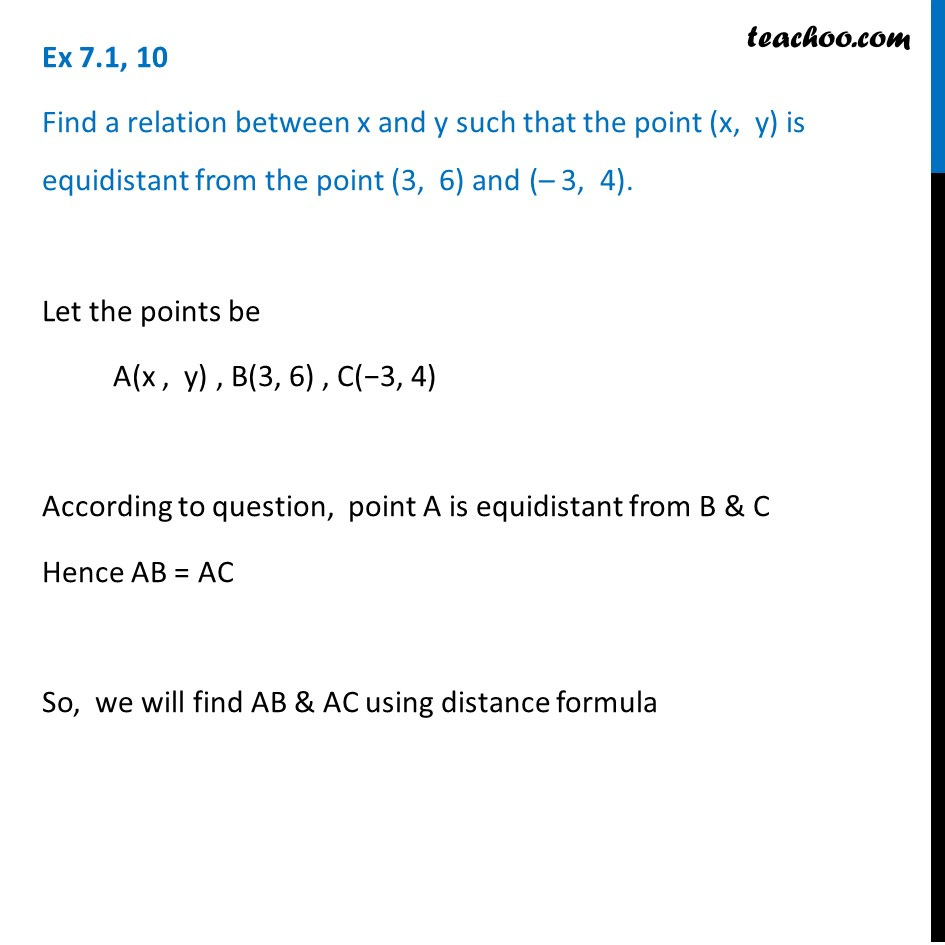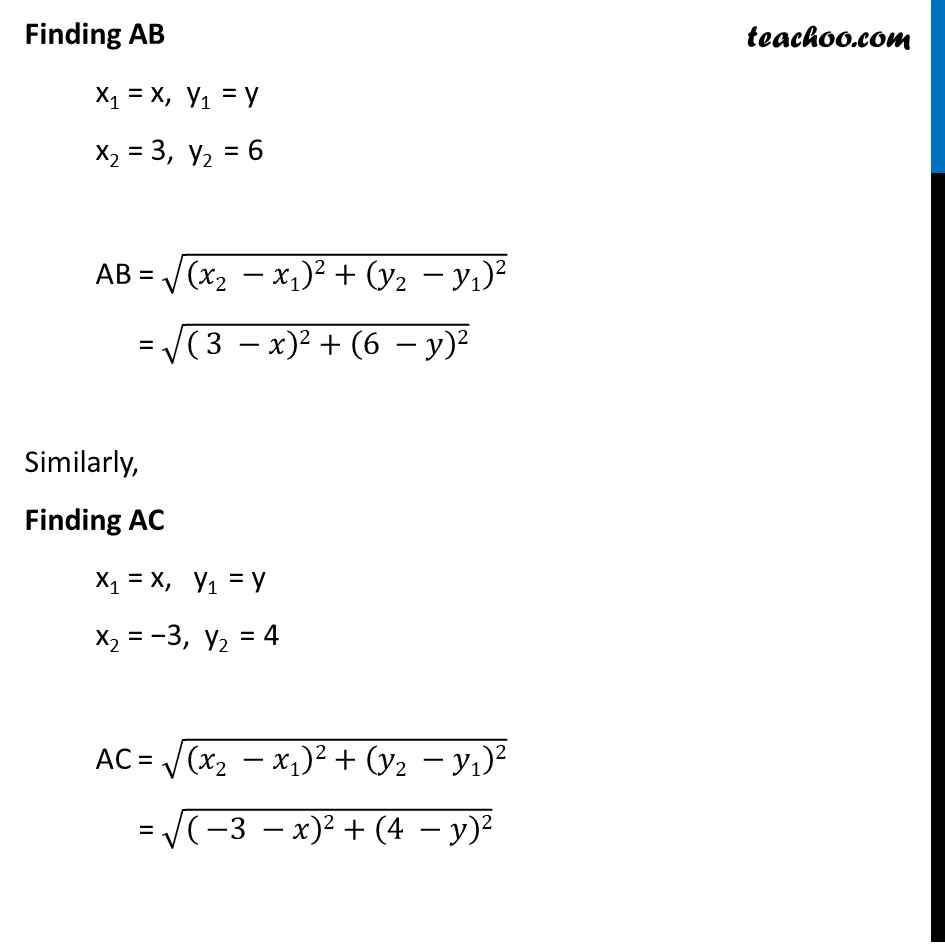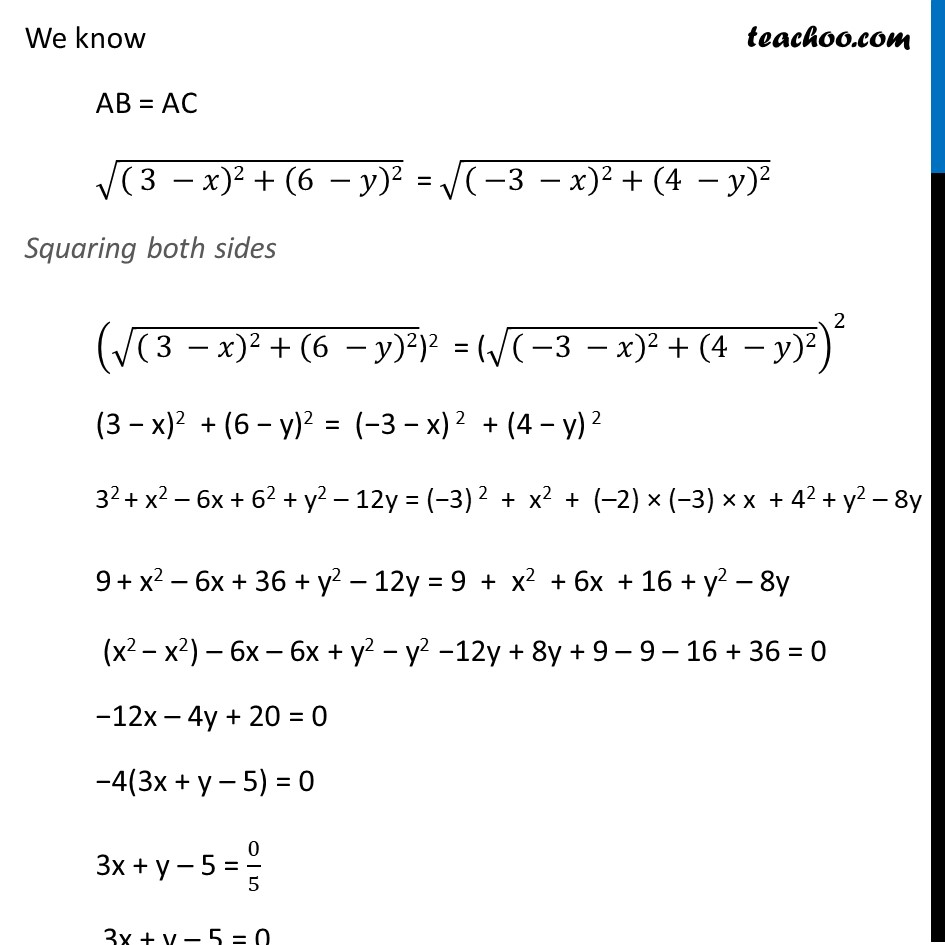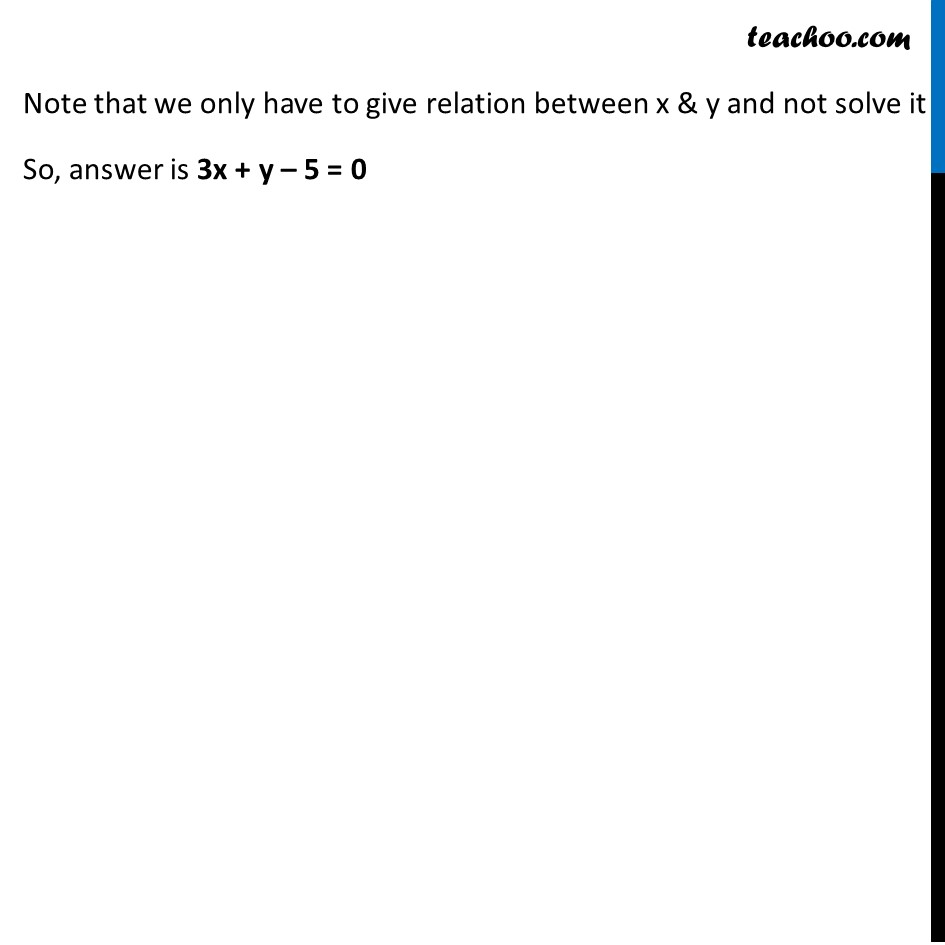Ex 7.1

Chapter 7 Class 10 Coordinate Geometry
Serial order wiseLearn in your speed, with individual attention - Teachoo Maths 1-on-1 Class

### Transcript

Ex 7.1, 10 Find a relation between x and y such that the point (x, y) is equidistant from the point (3, 6) and (– 3, 4). Let the points be A(x , y) , B(3, 6) , C(−3, 4) According to question, point A is equidistant from B & C Hence AB = AC So, we will find AB & AC using distance formula Finding AB x1 = x, y1 = y x2 = 3, y2 = 6 AB = √((𝑥2 −𝑥1)2+(𝑦2 −𝑦1)2) = √(( 3 −𝑥)2+(6 −𝑦)2) Similarly, Finding AC x1 = x, y1 = y x2 = −3, y2 = 4 AC = √((𝑥2 −𝑥1)2+(𝑦2 −𝑦1)2) = √(( −3 −𝑥)2+(4 −𝑦)2) We know AB = AC √(( 3 −𝑥)2+(6 −𝑦)2) = √(( −3 −𝑥)2+(4 −𝑦)2) Squaring both sides (√(( 3 −𝑥)2+(6 −𝑦)2) ")2 = (" √(( −3 −𝑥)2+(4 −𝑦)2))^2 (3 − x)2 + (6 − y)2 = (−3 − x) 2 + (4 − y) 2 32 + x2 – 6x + 62 + y2 – 12y = (−3) 2 + x2 + (–2) × (−3) × x + 42 + y2 – 8y 9 + x2 – 6x + 36 + y2 – 12y = 9 + x2 + 6x + 16 + y2 – 8y (x2 − x2) – 6x – 6x + y2 − y2 −12y + 8y + 9 – 9 – 16 + 36 = 0 −12x – 4y + 20 = 0 −4(3x + y – 5) = 0 3x + y – 5 = 0/5 3x + y – 5 = 0 Note that we only have to give relation between x & y and not solve it So, answer is 3x + y – 5 = 0